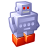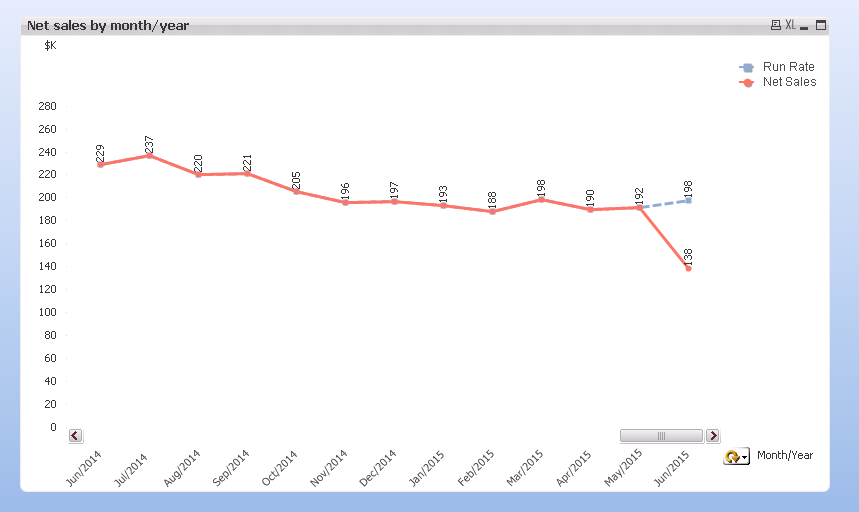# QlikView App Dev

Discussion Board for collaboration related to QlikView App Development.

Announcements
Join us for a live Q&A! September 21, 10 AM ET - Onboarding Fast in Qlik Sense SaaS! REGISTER
cancel
Showing results for
Did you mean:Specialist

## Last time value in time line chart

Hi,

I'm creating a time chart which presents sum of sales over time.

Time is actually a dimensional group: Month/Year, Year, Month, Day.

The example attached.

The problem is that in every last value of time dimension (like Jun/2015 if we choose Month/Year, or 2015 if we choose year etc)

the chart line goes drastically down which makes a totally wrong impression that the sales gone down lately.

What I want to do is to show sum of sales for all periods except for the last one, and for the last one I'd like to show sales RUN RATE.

And all this needs to be dynamic...

Thanks in advance for any assistance1 Solution

Accepted SolutionsMVP

Hi Linoy,

You need your current expression, and then add to it the run rate for the remaining days.  You can do this with a bit of set analysis.

First, try getting the current month added twice at the end.  To do this, set up a variable with the max MonthYear, call it vMaxMonthYear;

=Max(MonthYear)

The expression will then be something like;

sum(Value) + sum({<MonthYear={'\$(vMaxMonthYear)'}>}Value)

Check that works before moving on; you may need to put a Date function around the Max(MonthYear) to convert it to the correct format to match the month year.

Once you have that, you can set about turning it into a run rate.

You will want two more variables, vDaysInMaxMonth and vMaxDayInMaxMonth these will be something like;

=Day(MonthEnd(vMaxMonthYear))

And;

=Day(max({<MonthYear={'\$(vMaxMonthYear)'}>}Date))

Test that these variables have the correct values (eg. 30 and 21) before proceeding.

Now you want to work out the factor you need for your run rate from those values, a new variable vRunRateFactor can be set up:

=(vDaysInMaxMonth - vMaxDayInMaxMonth) / vMaxDayInMaxMonth

All being well, and if you get onto this today, you should get 0.3 in this variable.

You can then apply this factor back to your original expression:

sum(Value) + (sum({<MonthYear={'\$(vMaxMonthYear)'}>}Value) * vRunRateFactor)

Good spot that having a run rate like this is a "good thing".  If you have a bar chart, rather than a line chart, you can add the extra run rate on as a stacked bar (a separate expression) rather than adding on to the main expression - but you then lose the ability not to have an axis that doesn't start at zero.

You should make sure that somewhere on the chart that it is clear that the final month has a run rate added, perhaps with text in chart?

All code has been typed directly into this response, so may need a bit of tweaking, but hopefully it will be enough to point you in the right direction.

Hope that helps.

Steve

9 RepliesSpecialist III

you could use something like this

=if(max(Date) >= monthstart(date(max({1}Date))), 'expresion1','expresion2')Specialist
Author

Thanks Ramon, I tried it but what it does - it applies the expression for the whole range in chart and not only for the needed values...MVP

Hi Linoy,

You need your current expression, and then add to it the run rate for the remaining days.  You can do this with a bit of set analysis.

First, try getting the current month added twice at the end.  To do this, set up a variable with the max MonthYear, call it vMaxMonthYear;

=Max(MonthYear)

The expression will then be something like;

sum(Value) + sum({<MonthYear={'\$(vMaxMonthYear)'}>}Value)

Check that works before moving on; you may need to put a Date function around the Max(MonthYear) to convert it to the correct format to match the month year.

Once you have that, you can set about turning it into a run rate.

You will want two more variables, vDaysInMaxMonth and vMaxDayInMaxMonth these will be something like;

=Day(MonthEnd(vMaxMonthYear))

And;

=Day(max({<MonthYear={'\$(vMaxMonthYear)'}>}Date))

Test that these variables have the correct values (eg. 30 and 21) before proceeding.

Now you want to work out the factor you need for your run rate from those values, a new variable vRunRateFactor can be set up:

=(vDaysInMaxMonth - vMaxDayInMaxMonth) / vMaxDayInMaxMonth

All being well, and if you get onto this today, you should get 0.3 in this variable.

You can then apply this factor back to your original expression:

sum(Value) + (sum({<MonthYear={'\$(vMaxMonthYear)'}>}Value) * vRunRateFactor)

Good spot that having a run rate like this is a "good thing".  If you have a bar chart, rather than a line chart, you can add the extra run rate on as a stacked bar (a separate expression) rather than adding on to the main expression - but you then lose the ability not to have an axis that doesn't start at zero.

You should make sure that somewhere on the chart that it is clear that the final month has a run rate added, perhaps with text in chart?

All code has been typed directly into this response, so may need a bit of tweaking, but hopefully it will be enough to point you in the right direction.

Hope that helps.

SteveSpecialist
Author

Hi Steve,

All formulas were rightI added the run rate expression to the line chart I had and I got this:The only question is if it's possible to use run rate calculated the way you've suggested when I have a dimensional dates group (and not a single month/year dimension). For example, if a user wants to see Sales \$ by years or by days...

Do you think there is a way or I'll have to multiply the chart several times for each of the wanted dimensions?MVP

Hi Linoy,

Glad it worked out well for you.  The chart looks good.

If you want to change the dimension from Year to Month to Day you simply need to change the expression you are adding on.

If you are using a Drill Down group (which I wouldn't, see QlikView App: Dynamic Date Dimension) you can use GetCurrentField for this.  I presume for the day you don't need a run rate (unless you do a run rate to hours), you have the code for the month, and the year can be worked out in a similar fashion.

So, you would end up with:

sum(Value) +

if(GetCurrentField(DateDrilldown) = 'Year',

(sum({<Year={'\$(vMaxYear)'}>}Value) * vYearRunRateFactor)

if(GetCurrentField(DateDrilldown) = 'MonthYear',

(sum({<MonthYear={'\$(vMaxMonthYear)'}>}Value) * vRunRateFactor), 0))

The vYearRunRateFactor variable would need to know the day of the year, using DayNumberOfYear:

=DayNumberOfYear(max({<Year={'\$(vMaxYear)'}>}Date))

Hopefully you can work out the rest of the required values for vYearRunRateFactor from that?

Cheers,

SteveSpecialist
Author

Thanks a lot, Steve!!!

GetCurrentField didn't work in an expression, so I created a variable vGroup ='[' & GetCurrentField(Dates) & ']'

where Dates is my dimensional group.

My final expression is:

sum(ChargeTotal) +

sum({<\$(vGroup)={'Year'}, Year={'\$(vMaxYear)'}>}ChargeTotal * vYearRunRateFactor) +

sum({<\$(vGroup)={'MonthYear'}, MonthYear={'\$(vMaxMonthYear)'}>}ChargeTotal * vRunRateFactor)

I've read QlikView App: Dynamic Date Dimension - it's pretty cool.

I tried to change my dimension to a dynamic one as you suggest but I wasn't able to apply Run Rate calculations on it.

Is it possible to show Run Rate in this case as well?MVP

Hi Linoy,

On the back of this solution, I have created a LinkedIn post that further explores the advantages of calculating run rates:

I have also turned my solution above into a blog post on my web site:

http://www.quickintelligence.co.uk/qlik-run-rate/

If you look at the foot of the blog posting you will find links to downloadable examples for the solutions, with charts and code that can be copied and pasted.

Enjoy,

SteveMVP

Yes, you can.  Using your (rather neat) Set Analysis solution you simply need to change the vGroup variable to get it's value from the calculated dimension code, rather than the GetCurrentField function.Specialist
Author

Thank you, Steve!

You're amazingCommunity Browser